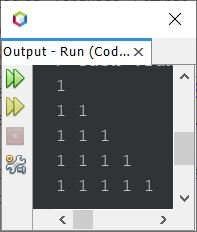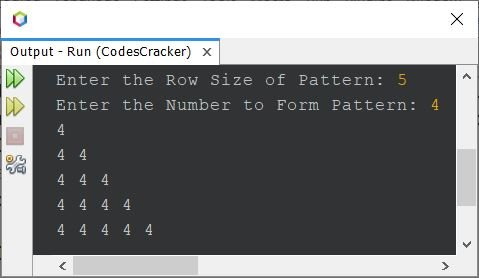# Java Program to Print Pattern of Numbers

This post covers all famous programs in Java that are used to print pattern of numbers. There are almost more than 7 pattern programs using numbers are created here.

## Number Pattern Program in Java - Pattern No.1

The question is, write a Java program to print pattern of number. Following program is its answer:

```public class CodesCracker
{
public static void main(String[] args)
{
int row=10, num=1;

for(int i=0; i<row; i++)
{
for(int j=0; j<=i; j++)
System.out.print(num+ " ");
System.out.print("\n");
}
}
}```

The snapshot given below shows the sample output of above Java program on printing of number pattern:### Print Number Pattern based on User Input

The previous program can also be created in a way to allow user to define the size of pattern along with the number to use, while forming the pattern:

```import java.util.Scanner;

public class CodesCracker
{
public static void main(String[] args)
{
int row, num;
Scanner s = new Scanner(System.in);

System.out.print("Enter the Row Size of Pattern: ");
row = s.nextInt();
System.out.print("Enter the Number to Form Pattern: ");
num = s.nextInt();

for(int i=0; i<row; i++)
{
for(int j=0; j<=i; j++)
System.out.print(num+ " ");
System.out.print("\n");
}
}
}```

The sample run of above program with user input 5 as row size and 4 as number, is shown in the snapshot given below:Note - You can use the same process to print the pattern of numbers based on user-input, in other programs given below.

## Number Pattern Program in Java - Pattern No.2

The program given below prints right-angled triangle of natural numbers. That is, 1 at first row, 2 3 at second row, 4 5 6 at third row, and so on:

```public class CodesCracker
{
public static void main(String[] args)
{
int r=10, num=1;

for(int i=0; i<r; i++)
{
for(int j=0; j<=i; j++)
{
System.out.print(num+ " ");
num++;
}
System.out.print("\n");
}
}
}```

#### Output of Previous Number Pattern Program

```1
2 3
4 5 6
7 8 9 10
11 12 13 14 15
16 17 18 19 20 21
22 23 24 25 26 27 28
29 30 31 32 33 34 35 36
37 38 39 40 41 42 43 44 45
46 47 48 49 50 51 52 53 54 55 ```

## Number Pattern Program in Java - Pattern No.3

Now this program prints pattern of numbers, where each row contains natural numbers. That is, 1 at first row, 1 2 at second row, 1 2 3 at third row, and so on.

```public class CodesCracker
{
public static void main(String[] args)
{
int r=10;

for(int i=0; i<r; i++)
{
int num=1;
for(int j=0; j<=i; j++)
{
System.out.print(num+ " ");
num++;
}
System.out.print("\n");
}
}
}```

#### Output of Previous Number Pattern Program

```1
1 2
1 2 3
1 2 3 4
1 2 3 4 5
1 2 3 4 5 6
1 2 3 4 5 6 7
1 2 3 4 5 6 7 8
1 2 3 4 5 6 7 8 9
1 2 3 4 5 6 7 8 9 10 ```

## Number Pattern Program in Java - Pattern No.4

This program also prints the same pattern as of previous, but this time, 1 at first row, 2 2 at second row, 3 3 3 at third row, and so on.

```public class CodesCracker
{
public static void main(String[] args)
{
int r=9, num=1;

for(int i=0; i<r; i++)
{
for(int j=0; j<=i; j++)
System.out.print(num+ " ");

System.out.print("\n");
num++;
}
}
}```

#### Output of Previous Number Pattern Program

```1
2 2
3 3 3
4 4 4 4
5 5 5 5 5
6 6 6 6 6 6
7 7 7 7 7 7 7
8 8 8 8 8 8 8 8
9 9 9 9 9 9 9 9 9 ```

## Number Pattern Program in Java - Pattern No.5

Now let me create some other pattern program of numbers in Java.

```public class CodesCracker
{
public static void main(String[] args)
{
int r=10, spaceLimit, num=1;
spaceLimit = (r*2) - 2;

for(int i=0; i<r; i++)
{
for(int space=0; space<spaceLimit; space++)
System.out.print(" ");
for(int j=0; j<=i; j++)
System.out.print(num+ " ");
System.out.print("\n");
spaceLimit = spaceLimit-2;
}
}
}```

#### Output of Previous Number Pattern Program

```                  1
1 1
1 1 1
1 1 1 1
1 1 1 1 1
1 1 1 1 1 1
1 1 1 1 1 1 1
1 1 1 1 1 1 1 1
1 1 1 1 1 1 1 1 1
1 1 1 1 1 1 1 1 1 1 ```

## Number Pattern Program in Java - Pattern No.6

```public class CodesCracker
{
public static void main(String[] args)
{
int r=10, num=1;

for(int i=0; i<r; i++)
{
for(int j=i; j<r; j++)
System.out.print(num+ " ");

System.out.print("\n");
}
}
}```

#### Output of Previous Number Pattern Program

```1 1 1 1 1 1 1 1 1 1
1 1 1 1 1 1 1 1 1
1 1 1 1 1 1 1 1
1 1 1 1 1 1 1
1 1 1 1 1 1
1 1 1 1 1
1 1 1 1
1 1 1
1 1
1 ```

## Number Pattern Program in Java - Pattern No.7

```public class CodesCracker
{
public static void main(String[] args)
{
int r=10, limit=0, num=1;

for(int i=0; i<r; i++)
{
for(int s=0; s<limit; s++)
System.out.print(" ");

for(int j=i; j<r; j++)
System.out.print(num+ " ");

System.out.print("\n");
limit += 2;
}
}
}```

#### Output of Previous Number Pattern Program

```1 1 1 1 1 1 1 1 1 1
1 1 1 1 1 1 1 1 1
1 1 1 1 1 1 1 1
1 1 1 1 1 1 1
1 1 1 1 1 1
1 1 1 1 1
1 1 1 1
1 1 1
1 1
1 ```

## Number Pattern Program in Java - Pattern No.8

This program is used to print equilateral triangle using a 1, a number.

```public class CodesCracker
{
public static void main(String[] args)
{
int r=10, num=1;

for(int i=0; i<r; i++)
{
for(int s=i; s<r; s++)
System.out.print(" ");

for(int j=0; j<=i; j++)
System.out.print(num+ " ");

System.out.print("\n");
}
}
}```

#### Output of Previous Number Pattern Program

```          1
1 1
1 1 1
1 1 1 1
1 1 1 1 1
1 1 1 1 1 1
1 1 1 1 1 1 1
1 1 1 1 1 1 1 1
1 1 1 1 1 1 1 1 1
1 1 1 1 1 1 1 1 1 1 ```

## Number Pattern Program in Java - Pattern No.9

```public class CodesCracker
{
public static void main(String[] args)
{
int r=10, num=1;

for(int i=0; i<r; i++)
{
for(int s=0; s<i; s++)
System.out.print(" ");

for(int j=i; j<r; j++)
System.out.print(num+ " ");

System.out.print("\n");
}
}
}```

#### Output of Previous Number Pattern Program

```1 1 1 1 1 1 1 1 1 1
1 1 1 1 1 1 1 1 1
1 1 1 1 1 1 1 1
1 1 1 1 1 1 1
1 1 1 1 1 1
1 1 1 1 1
1 1 1 1
1 1 1
1 1
1 ```

Note - For more pattern, refer to Star Pattern Program in Java. The only change, you need to do, is to change the star with number.

Java Online Test

« Previous Program Next Program »